# US8558939B2 - Image pickup lens and image pickup apparatus - Google Patents

## Info

Publication number
US8558939B2
US8558939B2 US13/501,678 US201013501678A US8558939B2 US 8558939 B2 US8558939 B2 US 8558939B2 US 201013501678 A US201013501678 A US 201013501678A US 8558939 B2 US8558939 B2 US 8558939B2
Authority
US
United States
Prior art keywords
lens
image pickup
image
surface facing
object side
Prior art date
Legal status (The legal status is an assumption and is not a legal conclusion. Google has not performed a legal analysis and makes no representation as to the accuracy of the status listed.)
Active
Application number
US13/501,678
Other versions
US20120206639A1 (en
Inventor
Kazuki Matsui
Yasunari Fukuta
Current Assignee (The listed assignees may be inaccurate. Google has not performed a legal analysis and makes no representation or warranty as to the accuracy of the list.)
Original Assignee
Priority date (The priority date is an assumption and is not a legal conclusion. Google has not performed a legal analysis and makes no representation as to the accuracy of the date listed.)
Filing date
Publication date
Priority claimed from JP2009239640 external-priority
Application filed by Konica Minolta Advanced Layers Inc filed Critical Konica Minolta Advanced Layers Inc
Assigned to KONICA MINOLTA ADVANCED LAYERS, INC. reassignment KONICA MINOLTA ADVANCED LAYERS, INC. ASSIGNMENT OF ASSIGNORS INTEREST (SEE DOCUMENT FOR DETAILS). Assignors: FUKUTA, YASUNARI, MATSUI, KAZUKI
Publication of US20120206639A1 publication Critical patent/US20120206639A1/en
Application granted granted Critical
Publication of US8558939B2 publication Critical patent/US8558939B2/en
Active legal-status Critical Current
Anticipated expiration legal-status Critical

• 230000014509 gene expression Effects 0.000 claims abstract description 114
• 230000005499 meniscus Effects 0.000 claims abstract description 68
• 230000003287 optical Effects 0.000 claims description 50
• 239000003779 heat-resistant material Substances 0.000 claims description 10
• 230000004075 alteration Effects 0.000 abstract description 152
• 238000010586 diagram Methods 0.000 description 86
• 201000009310 astigmatism Diseases 0.000 description 66
• 239000007787 solid Substances 0.000 description 40
• 239000011521 glass Substances 0.000 description 26
• 239000000758 substrate Substances 0.000 description 24
• 230000000694 effects Effects 0.000 description 16
• 206010010071 Coma Diseases 0.000 description 14
• 238000006243 chemical reaction Methods 0.000 description 14
• 238000004891 communication Methods 0.000 description 12
• 239000002131 composite material Substances 0.000 description 12
• 150000001875 compounds Chemical class 0.000 description 12
• 230000004907 flux Effects 0.000 description 12
• 210000001747 Pupil Anatomy 0.000 description 6
• 239000000463 material Substances 0.000 description 6
• 238000010276 construction Methods 0.000 description 4
• 230000000875 corresponding Effects 0.000 description 4
• 239000006059 cover glass Substances 0.000 description 4
• 239000004973 liquid crystal related substance Substances 0.000 description 4
• 230000002093 peripheral Effects 0.000 description 4
• 238000004904 shortening Methods 0.000 description 4
• 210000001138 Tears Anatomy 0.000 description 2
• 230000005540 biological transmission Effects 0.000 description 2
• 230000000295 complement Effects 0.000 description 2
• 230000003247 decreasing Effects 0.000 description 2
• 238000005516 engineering process Methods 0.000 description 2
• 229910044991 metal oxide Inorganic materials 0.000 description 2
• 150000004706 metal oxides Chemical class 0.000 description 2
• 238000000034 method Methods 0.000 description 2
• 239000005394 sealing glass Substances 0.000 description 2
• 239000004065 semiconductor Substances 0.000 description 2

## Images

•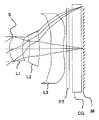•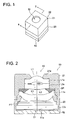•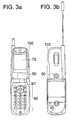•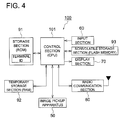•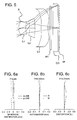•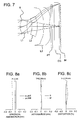•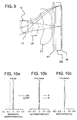•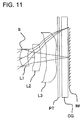••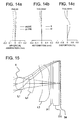•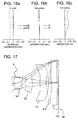•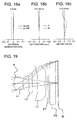•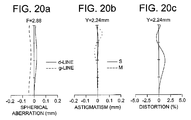•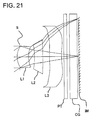•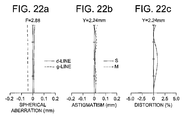•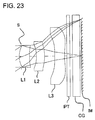•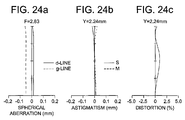## Classifications

• GPHYSICS
• G02OPTICS
• G02BOPTICAL ELEMENTS, SYSTEMS OR APPARATUS
• G02B13/00Optical objectives specially designed for the purposes specified below
• G02B13/001Miniaturised objectives for electronic devices, e.g. portable telephones, webcams, PDAs, small digital cameras
• G02B13/0015Miniaturised objectives for electronic devices, e.g. portable telephones, webcams, PDAs, small digital cameras characterised by the lens design
• G02B13/002Miniaturised objectives for electronic devices, e.g. portable telephones, webcams, PDAs, small digital cameras characterised by the lens design having at least one aspherical surface
• G02B13/0035Miniaturised objectives for electronic devices, e.g. portable telephones, webcams, PDAs, small digital cameras characterised by the lens design having at least one aspherical surface having three lenses

## Abstract

Provided is an image pickup lens composed of three lens elements and an image pickup apparatus using the same, where the image pickup lens has satisfactorily corrected aberrations despite being smaller in size than conventional image pickup lenses. As image pickup lens includes, in order from the object side, an aperture stop, a first lens, a second lens, and a third lens. The first lens is a positive lens and is a meniscus lens having a convex surface facing the object side and a concave surface facing the object side, and the third lens is a negative lens. The image pickup lens satisfies conditional expressions relating to an air gap between the second and third lenses, a focal length of the second lens, and a curvature radius of the object-side surface of the second lens.

## Description

RELATED APPLICATIONS
This is a U.S. National Phase Application under 35 USC 371 of International Application PCT/JP2010/067533 filed on Oct. 6, 2010.
This application claims the priority of JP 2009-239640 filed on Oct. 6, 2009, the entire content of which is hereby incorporated by reference.
TECHNICAL FIELD
The present invention relates to an image pickup lens suitable for an image pickup apparatus employing a solid-state image pickup element such as a CCD (Charge Coupled Devices) type image sensor and a CMOS (Complementary Metal-Oxide Semiconductor) type image sensor, and to an image pickup apparatus.
BACKGROUND ART
Recently, compact and thin image pickup apparatuses each employing a solid-state image pickup element such as a CCD type image sensor and a CMOS type image sensor have come to be mounted on mobile terminals being compact and thin electronic devices such as a cell phone and PDA (Personal Digital Assistant), and further on note PCs, which allows mutual transmission not only of sound information but also of image information to a remote location. Such the image pickup apparatuses are strongly demanded to be downsized and to handle high quality images realizing image quality expected by users.
A solid-state image pickup element and an image pickup lens are main components constructing an image pickup apparatus. As a solid-state image pickup element, many apparatuses use a device such as a CCD type image sensor and a CMOS type image sensor, In recent years, downsizing of pixel pitch of a solid-state image pickup element has been advanced, and high resolution and high performance are being achieved by using the increased number of pixels. On the other hand, downsizing of a solid-state image pickup element is being achieved by keeping the number of pixels.
In view of those, an image pickup lens which has a three-lens structure and includes a first lens and second lens both having positive power, has been proposed as disclosed in Patent Literature 1. Further, an image pickup lens wherein a second lens is negative has been proposed as shown in Patent literatures 2 to 4.
CITATION LIST
Patent Literature
Patent Literature 1: JP-A No. 2005-17884
Patent Literature 2: JP-A No. 2007-156277
Patent Literature 3: JP-B No. 4256442
Patent Literature 4: JP-A No. 2005-308800
SUMMARY OF INVENTION Technical Problem
However, in the image pickup lens disclosed in Patent Literature 1, correction of coma and astigmatism is not sufficient, and it is hardly considered that the image pickup lens is configured to handle an increased number of pixels of a solid-state image pickup element Further, it was difficult for image pickup lenses disclosed in Patent Literatures 2 to 4 to form high quality images, because of their insufficient F-numbers, insufficiently corrected astigmatism, and large curvature of field.
The present invention has been achieved in view of such the problems and is aimed to provide an image pickup lens which has a three-lens structure, and an image pickup apparatus using the image pickup lens. In the image pickup lens, various aberrations are corrected satisfactorily, while a smaller size than the conventional image pickup lenses is achieved.
As for a dimension of a small-sized image pickup lens, the present invention is aimed to achieve downsizing at the level satisfying the following expression (I).
TL/2Y′<0.9  (I)
In the expression, TL is a distance on the optical axis from the lens surface arranged at the closest position to the object side in the total system of the image pickup lens to the focal point at the image side, and 2Y′ is a diagonal length of an image pickup plane of a solid-state image pickup element (a diagonal length of a rectangular effective pixel area of the solid-state image pickup element). Satisfying the range allows to downsize the whole image pickup apparatus and reduce the outer diameter of the lens as a synergy effect. Thereby, the image pickup apparatus can be reduced in size and weight as a whole.
In the above description, “focal point at the image side” means an image point formed when a parallel ray which is parallel with the optical axis enters a lens. When there is an a parallel flat plate such as an optical low-pass filter, an infrared cut-off filter, a band-pass filter and a sealing glass of a solid-state image pickup element package, at a position between the lens surface arranged at the closest position to the image side in the image pickup lens and the focal point at the image side, the value of TL is calculated on the assumption that a space of the parallel flat plate is regarded as an air-equivalent distance.
Solution to Problem
An image pickup lens described as an aspect of the present invention is an image pickup lens characterized by comprising, in order from an object side thereof, an aperture stop, a first lens, a second lens and a third lens, wherein the first lens is a positive lens and is a meniscus lens having a convex surface facing the object side and a concave surface facing an image side, the second lens is a lens including a concave surface facing the object side, the third lens is a negative lens, and the image pickup lens satisfies the following conditional expressions.
0.10<D4/f<0.25  (1)
0.00≦|f/f2|<0.65  (2)
−2.50<f/r3<0.00  (3)
In the expressions, D4 is a distance (mm) of an air gap on an optical axis between the second lens and the third lens,
f is a focal length (mm) of a total system of the image pickup lens,
f2 is a focal length (mm) of the second lens, and
r3 is a curvature radius (mm) of the surface facing the object side of the second lens.
A basic construction of the present invention for obtaining a compact image pickup lens with excellently corrected aberrations, is composed of, in order from the object side, an aperture stop, a first lens having a positive refractive power and having a meniscus shape including a convex surface facing the object side and a concave surface facing the image side, a second lens including a concave surface facing the object side, and a third lens having negative refractive power. This lens construction is advantageous in downsizing the total length of the image pickup lens because the first surface is convex toward the object side and the second surface is concave toward the image side in the first lens. Further, the third lens being a negative lens makes the Petzval sum small, and enables to obtain an image pickup lens in which an excellent image-forming property is kept up to the periphery. By arranging an aperture stop at a position closer to the object side than the object-side surface of the first lens, the position of the exit pupil can be located farther than an image pickup plane. Therefore, the total length can be reduced, while telecentric property (hereinafter, is referred as “telecentricity”) is kept to be excellent
Herein, the relationship of the total length of the image pickup lens and the focal length of the first lens will be described. It is assumed that the lens constriction relating to the present invention is made up of a thin positive lens which is composed of the first lens and the second lens, and a thin negative lens which is composed of the third lens. The total length of the lens is provided by the following expression, where fl2 is a focal length of the first compound lens, d is a distance between the two compound lenses, fB is a distance (back focal length) from the second compound lens to the image plane.
$L = ⁢ fB + d = ⁢ f ⁡ ( 1 - d / f ⁢ ⁢ 12 ) + d = ⁢ f - ( ( f / ⁢ f ⁢ ⁢ 12 ) - 1 ) ⁢ d ( 7 )$
Assuming that the focal length f of the total system and the composite focal length fl2 of the first lens and second lens are constant, the expression (7) shows that the total length can be more reduced as the distanced between the compound lens composed of the first lens and the second lens, and the third lens is greater. On the other hand, assuming that the focal length f of the total system and distanced between the compound lens composed of the first lens and the second lens, and the third lens (namely, D4) are constant, the expression (7) shows that the total length can be more reduced as the composite focal length fl2 of the first lens and second lens is smaller.
(Effect of Conditional Expression (1))
Conditional expression (1) is a conditional expression for shortening the total length of the image pickup lens. As described above, in order to shorten the total length of the image pickup lens, distance D4 is preferably set to be broad. However, when the value of D4/f of Conditional expression (1) exceeds the upper limit, a bundle of axial rays entering the third lens becomes excessively thin and longitudinal chromatic aberration is hardly corrected in an excellent condition. Further, the height of off-axis rays entering the third lens becomes high and it makes the diameter of the third lens large, which is disadvantageous in making the lens compact On the other hand, when the value of D4/f of Conditional expression (1) is lower than the lower limit, the total lens length is hardly reduced effectively. Therefore, it is preferable that Conditional expression (1) is satisfied. Further, the range of the following expression (1′) is more preferable.
0.10<D4/f<0.20  (1′)
(Effects of Conditional Expression (2))
Conditional expression (2) is a conditional expression for shortening the total length of the image pickup lens. As described above, in order to shorten the total lens length, the composite focal length fl2 of the first lens and the second lens is required to be reduced. For reducing the composite focal length fl2, it can be considered to strengthen the power of the first lens firstly. However, an increase of the power of the first lens enlarges spherical aberration and coma to be generated at an off-axis area, and they are not corrected sufficiently with the succeeding lens.
When the power of the second lens is negative, the second lens with weaker power makes composite focal length fl2 smaller and makes the total length shorter. When the power of the second lens is positive, the second lens with greater power is advantageous in decreasing the total length, but excessively strong power of the second lens makes longitudinal chromatic aberration insufficiently corrected and further makes Petzval sum great so that curvature of field cannot be corrected. From the above viewpoints, it is preferable that the power of the second lens is weaker whether the power is positive or negative, for reducing the total length with correcting the longitudinal aberration and curvature of field. Therefore, it is preferable that Conditional expression (2) is satisfied. Further, the range of the following expression (2′) is more preferable.
0.00≦|f/f2|<0.55  (2′)
(Effects of Conditional Expression (3))
Conditional expression (3) is a conditional expression for correcting coma excellently at the intermediate image height with reducing the total length of the image pickup lens. For correcting coma, it is preferable to make curvature of a surface where a thick light flux passes as gentle as possible and to reduce the difference between curvature of the surface at a position around the optical axis of the light flux and curvature of the surface at a position around the periphery of the light flux. Therefore, it is preferable that Conditional expression (3) is satisfied. Further, the range of the following expression (3′) is more preferable.
−2.35<f/r3<0.00  (3′)
In the present specification, it is defined that the curvature radius of a convex surface is positive and the curvature radius of a concave surface is negative.
An image pickup lens as another aspect of the present invention is that the above image pickup lens is characterized in that at least a surface facing the image side of the third lens is an aspheric surface, and the aspheric surface includes at least one aspheric-surface inflection point.
By forming at least the surface facing the image side of the third lens into an aspheric shape including an inflection point, telecentric property of a light flux at the image side can be secured easily. Further, astigmatism and coma which are generated in an off-axis area can be corrected excellently. Herein, “an inflection point” means a point on an aspheric surface such that a tangential plane at a peak of the aspheric surface becomes perpendicular to the optical axis on a curve of the sectional shape of the lens within the effective radius.
An image pickup lens as another aspect of the present invention is that the above image pickup lens is characterized in that the third lens is a negative meniscus lens in which a convex surface faces the object side in a paraxial region thereof.
By forming the third lens into a meniscus shape whose convex surface faces the object side, the position of the principal point of the third lens being a negative lens is located closer to the image side, which enlarges distanced of the compound lens of the first lens and the second lens, and the third lens in the above expression (7), and enables to reduce the total lens length L.
An image pickup lens as another aspect of the present invention is that the above image pickup lens is characterized by satisfying the following expression (4).
−1.00<f3/f<0.00  (4)
In the expression, f3 is a focal length (mm) of the third lens.
(Effects of Conditional Expression (4))
Conditional expression (4) is a conditional expression for correcting various aberrations excellently with reducing the total length of the image pickup lens. The third lens as a negative lens having stronger power can keep longitudinal chromatic aberration and curvature of field smaller. However, the third lens having weaker power can keep telecentricity more excellently. Therefore, it is important to adjust the balance of them. When the value of f3/f becomes lower than the lower limit of Conditional expression (4), it is advantageous in aberration correction but the total length becomes excessively long, which is disadvantageous in downsizing. On the other hand, when the value of f3/f exceeds the upper limit of Conditional expression (4), aberration correction becomes insufficient. Therefore, it is preferable that the conditional expression (4) is satisfied.
An image pickup lens as another aspect of the present invention is that the above image pickup lens is characterized by satisfying the following expression (5).
−1.90<f/r4<0.40  (5)
In the expression, r4 is a curvature radius (mm) of a surface facing the image side of the second lens.
(Effects of Conditional Expression 5)
Conditional expression (5) is a conditional expression for correcting various aberrations excellently with reducing the total length of the image pickup lens. When the curvature of the object-side surface of the second lens is gentle, a lens in which Petzval sum and chromatic aberrations are corrected can be obtained, while the total length is kept to be small, by setting the value of r4 to satisfy Conditional expression (5). When the value of r4 is positive, the negative power of the second lens becomes greater as the value of r4 becomes smaller, which makes correction of various aberrations easy. However, when the value exceeds the upper limit of Conditional expression (5), the total length becomes excessively large. On the other hand, when the value of r4 is negative, the composite power of the first and second lenses becomes greater in the positive direction and the total length becomes shorter as the value of r4 becomes closer to zero. However, when the value becomes below the lower limit of Conditional expression (5), longitudinal chromatic aberration becomes great and Petzval sum is deteriorated. Therefore, it is preferable that the conditional expression (5) is satisfied.
An image pickup lens as another aspect of the present invention is that the above image pickup lens is characterized in that the second lens satisfies the following expression (6).
1.55<n2  (6)
In the expression, n2 is a refractive index of the second lens.
(Effects of Conditional Expression (6))
Conditional expression (6) is a conditional expression for correcting coma excellently with reducing the total length of the image pickup lens. By increasing the refractive index of the second lens so as to satisfy Conditional expression (6), the curvature radius of the object-side surface of the second lens can be furthermore reduced, and coma can be corrected excellently.
An image pickup lens as another aspect of the present invention is that the above image pickup lens is characterized in that each of the first lens, the second lens and the third lens is formed of a heat-resistant material.
By forming each of the first lens, the second lens and the third lens out of a heat-resistant material, they can be used for a so-called reflow processing that electric parts and lens modules are mounted on a substrate at the same time in a high-temperature reflow furnace. Therefore, a large number of image pickup apparatuses can be produced at low cost The heat-resistant material is preferably a heat-resistant glass.
An image pickup apparatus as another aspect of the present invention is characterized by comprising any one of the above image pickup lenses and a solid-state image pickup element.
According to the present invention, there can be provided a three-element image pickup lens which is smaller than the conventional image pickup lenses and exhibits excellently corrected various aberrations, and an image pickup apparatus equipped with the image pickup lens.
BRIEF DESCRIPTION OF DRAWINGS
FIG. 1 shows a perspective view illustrating an image pickup apparatus 50 relating to the present embodiment.
FIG. 2 shows a sectional view illustrating the structure of FIG. 1 which is cut along arrowed lines II-II, and is viewed in the direction of the arrows.
Each of FIGS. 3 a and 3 b shows a condition that image pickup apparatus 50 is mounted on cell phone 100 as a mobile terminal.
FIG. 4 shows a control block diagram of cell phone 100.
FIG. 5 shows a sectional view of the image pickup lens of Example 1.
FIGS. 6 a, 6 b, and 6 c show aberration diagrams of spherical aberration, astigmatism, and distortion of the image pickup lens of Example 1.
FIG. 7 shows a sectional view of the image pickup lens of Example 2.
FIG. 8 a, 8 b, and 8 c show aberration diagrams of spherical aberration, astigmatism, and distortion of the image pickup lens of Example 2.
FIG. 9 shows a sectional view of the image pickup lens of Example 3.
FIGS. 10 a, 10 b, and 10 c show aberration diagrams of spherical aberration, astigmatism, and distortion of the image pickup lens of Example 3.
FIG. 11 shows a sectional view of the image pickup lens of Example 4.
FIGS. 12 a, 12 b, and 12 c show aberration diagrams of spherical aberration, astigmatism, and distortion of the image pickup lens of Example 4.
FIG. 13 shows a sectional view of the image pickup lens of Example 5.
FIGS. 14 a, 14 b, and 14 c show aberration diagrams of spherical aberration, astigmatism, and distortion of the image pickup lens of Example 5.
FIG. 15 shows a sectional view of the image pickup lens of Example 6.
FIGS. 16 a, 16 b, and 16 c show aberration diagrams of spherical aberration, astigmatism, and distortion of the image pickup lens of Example 6.
FIG. 17 shows a sectional view of the image pickup lens of Example 7.
FIGS. 18 a, 18 b, and 18 c show aberration diagrams of spherical aberration, astigmatism, and distortion of the image pickup lens of Example 7.
FIG. 19 shows a sectional view of the image pickup lens of Example 8.
FIGS. 20 a, 20 b, and 20 c show aberration diagrams of spherical aberration, astigmatism, and distortion of the image pickup lens of Example 8.
FIG. 21 shows a sectional view of the image pickup lens of Example 9.
FIGS. 22 a, 22 b, and 22 c show aberration diagrams of spherical aberration, astigmatism, and distortion of the image pickup lens of Example 9.
FIG. 23 shows a sectional view of the image pickup lens of Example 10.
FIGS. 24 a, 24 b, and 24 c show aberration diagrams of spherical aberration, astigmatism, and distortion of the image pickup lens of Example 10.
DESCRIPTION OF EMBODIMENTS
The embodiment of the present invention will be described with referring to the drawings. FIG. 1 is a perspective view of image pickup apparatus 50 relating to the present embodiment. FIG. 2 is a sectional view illustrating the structure of FIG. 1 which is cut along arrowed lines II-II and is viewed in the arrowed direction. As shown in FIG. 2, image pickup apparatus 50 includes CMOS type image sensor 51 as a solid-state image pickup element having photoelectric conversion section 51 a; image pickup lens 10 for forming a subject image onto photoelectric conversion section 51 a of the image sensor 51; substrate 52 supporting image sensor 51 and including an external connecting terminal (which is unillustrated) for transmitting and receiving electric signal of the image sensor 51. They are integrally formed in one body.
The above image sensor 51 includes photoelectric conversion section 51 a representing a light-receiving section, on the central portion of a surface on the light-receiving side of the image sensor 51, and is connected to a signal processing circuit which is not illustrated, wherein on the photoelectric conversion section 51 a, pixels (photoelectric conversion elements) are arranged on a two-dimensional basis. The signal processing circuit is composed of a drive circuit section that obtains signal electric charges by driving respective pixels in succession, A/D converting section that converts each signal electric charge into digital signal and of a signal processing section that forms an output of image signal by using the digital signal. Around the outer edge of the light-receiving-side plane of image sensor 51, many pads (which are not illustrated) are provided and connected with substrate 52 through wires which are not illustrated. The image pickup element 51 converts the signal charges from the photoelectric conversion section 51 a into image signal such as digital YUV signal, and outputs it to a predetermined circuit on substrate 52 through wires (which are not illustrated). Reference sign Y represents luminance signal, U (=R−Y) represents color difference signal between red and the luminance signal, and V (=B−Y) represents color difference signal between blue and the luminance signal. Hereupon, the solid-state image pickup element is not limited to the above-described CMOS type image sensor, but another element such as a CCD can be employed.
Substrate 52 supporting image sensor 51 is connected to image sensor 51 with wires which are not illustrated so as to enable communication between them.
Substrate 52 is connected with an external circuit (for example, a control circuit provided by the higher level of apparatus of a mobile terminal on which the image pickup apparatus is mounted) through an unillustrated external connecting terminal, which enables to receive voltage and clock signal for driving image sensor 51 from the external circuit and to output the digital YUV signal to the external circuit.
The top of image sensor 51 is covered and sealed with cover glass CG which is attached to lower lens frame 20 in a rectangular tube (or a cylindrical) shape. Further, above cover glass CG, plate PT such as an infrared cut-off filter is fixed to lower lens frame 20. The lower end of lower lens frame 20 holding the edges of glass cover CG and plate PT is fixed to the upper surface of substrate 52 with surrounding image sensor 51. The upper end of lower lens frame 20 is connected with the lower and of upper lens frame 21 with being fitted into the upper lens frame. Upper lens frame 21 is hollow and includes flange section 21 a extending inside in the direction perpendicular to the optical axis, on its upper end. Image pickup lens 10 is arranged inside the flange section with being fitted to the flange section. Image pickup lens 10 is composed of, in order from the object side (upward in FIG. 2), aperture stop formed by an opening of flange section 21 a, first lens L1 having positive refractive power and having a meniscus shape with a convex surface facing the object side and a concave surface facing the image side, second lens L2 including a concave surface facing the object side, and third lens L3 having negative refractive power. Each of lenses L1 to L3 is preferably formed of a heat-resistant material, for example a glass material.
The top surface of flange section L1 a of first lens L1 comes in contact with the bottom surface of flange section 21 a of upper lens frame 21. The top surface of flange section L2 a of second lens L2 comes in contact with the bottom surface of flange section L1 a of first lens L1 directly with supporting light-shielding member SH in a ring plate shape between the surfaces. The bottom surface of flange section L2 a of second lens L2 comes in contact with the top surface of fixing member SP which is attached to upper lens frame 20, has a ring plate shape and doubles as a spacer. The top surface of flange section L3 a, of third lens L3 comes in contact with the bottom surface of the fixing member SP. Thereby, distances between lenses can be adjusted correctly. At the center of flange section 21 a of upper lens frame 21, as shown in the figure, an aperture stop in a circular shape is formed. The object-side optical surface of first lens L1 protrudes from the aperture stop toward the object side.
In the present embodiment, the following expressions (1) to (3) hold, where D4 is a distance (mm) of an air gap on an optical axis between second lens L2 and third lens L3, f is a focal length (mm) of the total system of the image pickup lens, f2 is a focal length (mm) of second lens L2, and r3 is a curvature radius (mm) of the surface facing the object side of the second lens.
0.10<D4/f<0.25  (1)
0.00≦|f/f2<0.65  (2)
−2.50<f/r3<0.00  (3)
In an assembling process, lower lens frame 20 to which plate PT has been joined is joined and bonded to substrate 52 so as to cover CMOS type image sensor 51 which has been arranged on substrate 52, and upper lens frame 21 in which first lens L1, light-shielding member SH, second lens L2, fixing member SP, third lens L3 are inserted in this order is bonded to lower lens frame 20. However, the assembling embodiment is not limited to the above. After lower lens frame 20 to which plate PT has been attached and upper lens frame 21 in which first lens L1, light-shielding member SH, second lens L2, fixing member SP, third lens L3 have been inserted in this order are bonded together, the resulting body may be bonded to substrate 52 so as to cover CMOS type image sensor 51 which has been arranged on substrate 52.
Next, a utilization embodiment of the above-described image pickup apparatus 50 will be described. Each of FIGS. 3 a and 3 b is a diagram showing a condition that image pickup apparatus 50 is mounted on cell phone 100 as a mobile terminal. FIG. 4 is a control block diagram of cell phone 100.
In image pickup apparatus 50, as shown in FIGS. 3 a and 3 b, for example, the object-side end surface of the image pickup lens is arranged on the rear surface (where it is assumed that the side of liquid crystal display section is the front) of cell phone 100, so as to be located at a position corresponding to an area below the liquid crystal display section. The arrangement of image pickup apparatus 50 is not limited to that.
The external connecting terminal (which is unillustrated) of image pickup apparatus 50 is connected with control section 101 of cell phone 100 to output image signals such as luminance signal and color difference signal to control section 101.
On the one hand, as shown in FIG. 4, cell phone 100 is provided with: control section (CPU) 101 which centrally controls respective sections and executes programs corresponding to various processing, input section 60 for indicating and inputting information such as number; display section 70 for displaying predetermined data and picked-up images; radio communication section 80 for realizing various kinds of information communication to an external server; storage section (ROM) 91 which stores system programs of the cell phone 100, various processing programs, and necessary data such as terminal ID; and temporary storage section (RAM) 92 which temporarily stores various processing programs and data to be processed by control section 101, processed data, and image data from the image pickup apparatus 50 and is used as a working area.
Image signal inputted from image pickup apparatus 50 is stored in nonvolatile storage section (flash memory) 93, is displayed on display section 70, or is transmitted to the outside as image information through radio communication section 80, by the control section 101 of cell phone 100. Further, cell phone 100 includes a microphone and speaker for inputting and outputting voices, which are not illustrated.
When a photographer holding cell phone 100 aims image pickup lens 10 of image pickup apparatus 50 at the photographic subject, image signal of a still image or movie is captured in image sensor 51. When the photographer pushes button BT in input section 60 shown in FIG. 3 a at the desired timing of shooting, the shutter is released, which results in capturing image signal in image pickup apparatus 50. The image signal inputted in image pickup apparatus 50 is transmitted to the control section in the above-described cell phone 100, to be stored in nonvolatile storage section 93, be displayed in display section 70 or be transmitted outside as image information through radio communication section 80.
EXAMPLES
Next, examples suitable for the above-described embodiment will be described. However, the scope of the invention is not limited to the examples which will be described below. Symbols used in each example represent the followings.
f. Focal length of the total system of the image pickup lens
fB: Back focal length
F: F-number
2Y: Diagonal length of the image pickup plane of the solid-state image pickup element
D: Surface distance on the optical axis
Nd: Refractive index of a lens material for d-line
vd: Abbe number of a lens material
ENTP: Position of the entrance pupil under the condition that the object-side surface of the first lens is assumed to be the basis
EXTP: Position of the exit pupil under the condition that the rearmost surface of the image pickup optical system is assumed to be the basis
H1: Position of the front principal point
H2: Position of the tear principal point
In each example, a surface represented by a surface number followed by an asterisk “*” is a surface having an aspheric shape. The shape of the aspheric surface is expressed by the following Math. 1, where the peak of the surface is defined as the origin, X-axis extends along the optical axis direction, and h represents the height in a direction perpendicular to the optical axis. In the following descriptions (including data of Tables), the power of 10 (for example, 2.5×10−02) will be expressed as by using “E” (for example, 2.5E-02). A blank in numerical data in Tables represents zero.
$X = h 2 / R 1 + 1 - ( 1 + K ) ⁢ h 2 / R 2 + ∑ A i ⁢ h i [ Math . ⁢ 1 ]$
In the expression, Ai is i-th-order aspheric surface coefficient, R is a curvature radius, and K is a conic constant.
Example 1
Table 1 shows lens data of Example 1. FIG. 5 shows a sectional view of the image pickup lens of Example 1. In the figure, S represents an aperture stop, L1 represents the first lens having positive refractive power and having a meniscus shape with a convex surface facing the object side and a concave surface facing the image side, L2 represents the second lens having negative refractive power and having a meniscus shape with a concave surface facing the object side and a convex surface facing the image side, L3 represents the third lens having negative refractive power, having a meniscus shape with a convex surface facing the object side, and including an inflection point on each of the object-side surface and the image-side surface, PT represents a parallel flat plate which is assumed to be a component such as an optical low-pass filter and IR cut-off filter, CG represents a parallel flat plate which is assumed to be a component such as a seal glass of a solid-state image pickup element, and IM represents a solid-state image pickup element. FIGS. 6 a, 6 b, and 6 c show aberration diagrams of spherical aberration, astigmatism, and distortion of the image pickup lens of Example 1. In the spherical aberration diagram, a dashed line and a solid line represent the amounts of spherical aberration for g-line and d-line, respectively. In the astigmatism diagram, the solid line represents a sagittal plane and the dashed line represents a meridional plane.
 TABLE 1 Example 1
 f = 3.74 mm fB = 0.12 mm F = 2.84 2Y = 4.48 mm ENTP = 0 mm EXTP = −2.36 mm H1 = −1.9 mm H2 = −3.62 mm
 Surface Effective No. R(mm) D(mm) Nd νd radius (mm) 1 ∞ 0.05 0.65 2 ∞ −0.23 0.65 3* 1.054 0.72 1.58020 56.3 0.67 4* 2.027 0.39 0.61 5* −3.116 0.55 1.80470 24.3 0.70 6* −4.835 0.63 0.95 7* 3.618 0.70 1.58020 56.3 1.52 8* 2.161 0.19 1.85 9 ∞ 0.15 1.51390 60.7 2.09 10 ∞ 0.18 2.12 11 ∞ 0.50 1.46920 61.7 2.19 12 ∞ 0.12 2.33
 *: Aspheric surface coefficient
 Third surface K = −0.14294E+00 A4 = 0.38162E−01 A6 = 0.63170E−01 A8 = −0.20792E−01 A10 = 0.18432E+00 A12 = −0.48270E−01 A14 = 0.94529E−01 Fourth surface K = 0.80226E+01 A4 = 0.74974E−02 A6 = 0.64686E−01 A8 = 0.19354E+00 A10 = −0.50703E+00 A12 = −0.34529E+00 A14 = 0.59938E+01 Fifth surface K = −0.49984E+02 A4 = −0.31608E+00 A6 = 0.34755E+00 A8 = −0.17102E+00 A10 = −0.41506E+00 A12 = −0.13420E+01 A14 = 0.50594E+01 A16 = −0.27483E+01 Sixth surface K = 0.16674E+01 A4 = −0.79727E−01 A6 = 0.13899E+00 A8 = −0.44057E−01 A10 = −0.19369E−01 A12 = 0.16993E−01 A14 = 0.93716E−01 A16 = −0.80329E−01 Seventh surface K = −0.44874E+02 A4 = −0.14756E+00 A6 = 0.35194E−01 A8 = −0.32262E−02 A10 = 0.18592E−02 A12 = 0.36658E−03 A14 = −0.16039E−03 A16 = −0.31846E−04 Eighth surface K = −0.10719E+02 A4 = −0.96568E−01 A6 = 0.26771E−01 A8 = −0.11261E−01 A10 = 0.19879E−02 A12 = 0.15579E−03 A14 = −0.12433E−03 A16 = 0.15264E−04
 Single lens data
 Lens block Forefront surface Focal length (mm) 1 3 2.976 2 5 −12.700 3 7 −11.228
Example 2
Table 2 shows lens data of Example 2. FIG. 7 shows a sectional view of the image pickup lens of Example 2. In the figure, S represents an aperture stop, L1 represents the first lens having positive refractive power and having a meniscus shape with a convex surface facing the object side and a concave surface facing the image side, L2 represents the second lens having positive refractive power and having a meniscus shape with a concave surface facing the object side and a convex surface facing the image side, L3 represents the third lens having negative refractive power, having a meniscus shape with a convex surface facing the object side, and including an inflection point on each of the object-side surface and the image-side surface, PT represents a parallel flat plate which is assumed to be a component such as an optical low-pass filter and IR cut-off filter, CG represents a parallel flat plate which is assumed to be a component such as a seal glass of a solid-state image pickup element, and IM represents a solid-state image pickup element. FIGS. 8 a, 8 b, and 8 c show aberration diagrams of spherical aberration, astigmatism, and distortion of the image pickup lens of Example 2. In the spherical aberration diagram, a dashed line and a solid line represent the amounts of spherical aberration for g-line and d-line, respectively. In the astigmatism diagram, the solid line represents a sagittal plane and the dashed line represents a meridional plane.
 TABLE 2 Example 2
 f = 3.74 mm fB = 0.13 mm F = 2.84 2Y = 4.48 mm ENTP = 0 mm EXTP = −2.38 H1 = −1.85 mm H2 = −3.61 mm mm
 Surface Effective No. R(mm) D(mm) Nd νd radius (mm) 1 ∞ 0.05 0.65 2 ∞ −0.21 0.65 3* 1.127 0.69 1.58020 56.3 0.66 4* 2.201 0.52 0.64 5* −2.280 0.55 1.58020 56.3 0.76 6* −2.474 0.61 1.00 7* 3.534 0.61 1.58020 56.3 1.51 8* 1.780 0.20 1.84 9 ∞ 0.15 1.51390 60.7 2.08 10 ∞ 0.18 2.12 11 ∞ 0.50 1.46920 61.7 2.19 12 ∞ 0.13 2.33
 Aspheric surface coefficient
 Third surface K = −0.16065E+00 A4 = 0.36307E−01 A6 = 0.73927E−01 A8 = −0.60479E−01 A10 = 0.13803E+00 A12 = 0.16821E+00 A14 = −0.19006E+00 Fourth surface K = 0.65088E+01 A4 = 0.43977E−01 A6 = 0.70312E−01 A8 = 0.25260E+00 A10 = 0.24832E+00 A12 = −0.19076E+01 A14 = 0.57815E+01 Fifth surface K = −0.14935E+02 A4 = −0.28182E+00 A6 = 0.14865E+00 A8 = 0.60631E−01 A10 = 0.26416E+00 A12 = −0.17018E+01 A14 = 0.35440E+01 A16 = −020804E+01 Sixth surface K = −0.20549E+01 A4 = −0.13239E+00 A6 = 0.13404E+00 A8 = 0.13421E−01 A10 = −0.36910E−01 A12 = 0.30183E−01 A14 = 0.10028E+00 A16 = −0.82484E−01 Seventh surface K = −0.42140E+02 A4 = −0.22333E+00 A6 = 0.74301E−01 A8 = −0.10163E−01 A10 = −0.51029E−03 A12 = 0.19621E−02 A14 = −0.15602E−03 A16 = −0.11872E−03 Eighth surface K = −0.10411E+02 A4 = −0.11511E+00 A6 = 0.32495E−01 A8 = −0.10621E−01 A10 = 0.16396E−02 A12 = 0.15521E−03 A14 = −0.12966E−03 A16 = 0.18035E−04
 Single lens data
 Lens block Forefront surface Focal length (mm) 1 3 3.219 2 5 1191.466 3 7 −7.087
Example 3
Table 3 shows lens data of Example 3. FIG. 9 shows a sectional view of the image pickup lens of Example 3. In the figure, S represents an aperture stop, L1 represents the first lens having positive refractive power and having a meniscus shape with a convex surface facing the object side and a concave surface facing the image side, L2 represents the second lens having negative refractive power and having a meniscus shape with a concave surface facing the object side and a convex surface king the image side, L3 represents the third lens having negative refractive power, including concave surfaces facing the object side and the image side, and including an inflection point on the image-side surface, PT represents a parallel flat plate which is assumed to be a component such as an optical low-pass filter and IR cut-off filter, CG represents a parallel flat plate which is assumed to be a component such as a seal glass of a solid-state image pickup element, and IM represents a solid-state image pickup element. FIGS. 10 a, 10 b, and 10 c show aberration diagrams of spherical aberration, astigmatism, and distortion of the image pickup lens of Example 3. In the spherical aberration diagram, a dashed line and a solid line represent the amounts of spherical aberration for g-line and d-line, respectively. In the astigmatism diagram, the solid line represents a sagittal plane and the dashed line represents a meridional plane.
 TABLE 3 Example 3
 f = 3.75 mm fB = 0.06 mm F = 2.85 2Y = 4.48 mm ENTP = 0 mm EXTP = −2.27 H1 = −2.35 mm H2 = −3.72 mm mm
 Surface Effective No. R(mm) D(mm) Nd νd radius (mm) 1 ∞ 0.00 0.66 2 ∞ −0.22 0.66 3* 1.074 0.67 1.58310 59.5 0.66 4* 3.238 0.51 0.66 5* −1.618 0.50 1.80540 26.1 0.65 6* −2.209 0.56 0.93 7* −11.957 0.77 1.58310 59.5 1.47 8* 3.535 0.15 1.85 9 ∞ 0.15 1.51630 64.1 2.50 10 ∞ 0.12 2.50 11 ∞ 0.50 1.51630 64.1 2.50 12 ∞ 0.06 2.50
 Aspheric surface coefficient
 Third surface K = 0.77576E−01 A4 = −0.43734E−02 A6 = −0.57588E−01 A8 = 0.19682E+00 A10 = −0.44927E+00 A12 = 0.22994E+00 Fourth surface K = 0.17855E+02 A4 = −0.75404E−01 A6 = −0.16731E+00 A8 = −0.93226E−01 A10 = −0.17365E+00 A12 = −0.14604E+01 Fifth surface K = −0.32941E+01 A4 = −0.35185E+00 A6 = 0.55436E+00 A8 = −0.60869E+01 A10 = 0.20013E+02 A12 = −0.27998E+02 Sixth surface K = 0.64933E+00 A4 = −0.32956E−01 A6 = −0.19114E−02 A8 = 0.17176E+00 A10 = −0.47875E−01 A12 = 0.14935E−01 Seventh surface K = −0.20704E+02 A4 = −0.19701E+00 A6 = 0.59140E−01 A8 = 0.15397E−01 A10 = −0.20960E−02 A12 = −0.27374E−02 A14 = 0.53450E−03 Eighth surface K = −0.32968E+02 A4 = −0.82174E−01 A6 = 0.31362E−02 A8 = −0.17062E−03 A10 = 0.14061E−02 A12 = −0.76479E−03 A14 = 0.10113E−03
 Single lens data
 Lens block Forefront surface Focal length (mm) 1 3 2.471 2 5 −12.072 3 7 −4.594
Example 4
Table 4 shows lens data of Example 4. FIG. 11 shows a sectional view of the image pickup lens of Example 4. In the figure, S represents an aperture stop, L1 represents the first lens having positive refractive power and having a meniscus shape with a convex surface facing the object side and a concave surface facing the image side, L2 represents the second lens having negative refractive power and having a meniscus shape with a concave surface facing the object side and a convex surface facing the image side, L3 represents the third lens having negative refractive power, having a meniscus shape with a convex surface facing the object side, and including an inflection point on each of the object-side surface and the image-side surface, PT represents a parallel flat plate which is assumed to be a component such as an optical low-pass filter and IR cut-off filter, CG represents a parallel flat plate which is assumed to be a component such as a seal glass of a solid-state image pickup element, and IM represents a solid-state image pickup element. FIGS. 12 a, 12 b, and 12 c show aberration diagrams of spherical aberration, astigmatism, and distortion of the image pickup lens of Example 4. In the spherical aberration diagram, a dashed line and a solid line represent the amounts of spherical aberration for g-line and d-line, respectively. In the astigmatism diagram, the solid line represents a sagittal plane and the dashed line represents a meridional plane.
 TABLE 4 Example 4
 f = 3.71 mm fB = 0.12 mm F = 2.88 2Y = 4.48 mm ENTP = 0 mm EXTP = −2.29 mm H1 = −2 mm H2 = −3.59 mm
 Surface Effective No. R(mm) D(mm) Nd νd radius (mm) 1 ∞ 0.05 0.64 2 ∞ −0.22 0.64 3* 1.046 0.64 1.58020 56.3 0.64 4* 2.349 0.55 0.62 5* −2.623 0.50 1.80470 24.3 0.68 6* −5.254 0.45 0.99 7* 3.543 0.77 1.58020 56.3 1.51 8* 2.227 0.18 1.85 9 ∞ 0.15 1.51390 60.7 3.00 10 ∞ 0.18 3.00 11 ∞ 0.50 1.52040 52.0 3.00 12 ∞ 0.12 3.00
 Aspheric surface coefficient
 Third surface K = −0.18419E+00 A4 = 0.30273E−01 A6 = 0.74560E−01 A8 = −0.65954E−01 A10 = 0.81976E−01 A12 = 0.86558E−01 A14 = 0.18458E+00 Fourth surface K = 0.10553E+02 A4 = −0.24998E−01 A6 = −0.13800E+00 A8 = 0.38914E+00 A10 = −0.68935E+00 A12 = −0.26705E+01 A14 = 0.42813E+01 Fifth surface K = −0.32944E+02 A4 = −0.39851E+00 A6 = 0.17092E+00 A8 = −0.74197E+00 A10 = 0.18564E+00 A12 = 0.20367E+01 A14 = −0.23722E+01 A16 = −0.10792E+02 Sixth surface K = 0.12434E+02 A4 = −0.11677E+00 A6 = 0.71559E−01 A8 = 0.31016E−02 A10 = 0.20201E−01 A12 = −0.14425E−01 A14 = −0.17903E−01 A16 = 0.24104E−01 Seventh surface K = −0.50000E+02 A4 = −0.17378E+00 A6 = 0.39679E−01 A8 = 0.44360E−02 A10 = 0.11508E−02 A12 = −0.17953E−03 A14 = −0.31225E−03 A16 = 0.36588E−04 Eighth surface K = −0.15765E+02 A4 = −0.85782E−01 A6 = 0.96683E−02 A8 = −0.14974E−02 A10 = 0.13844E−03 A12 = −0.18312E−04 A14 = −0.41008E−04 A16 = 0.10859E−04
 Single lens data
 Lens block Forefront surface Focal length (mm) 1 3 2.755 2 5 −7.111 3 7 −13.160
Example 5
Table 5 shows lens data of Example 5. FIG. 13 shows a sectional view of the image pickup lens of Example 5. In the figure, S represents an aperture stop, L1 represents the first lens having positive refractive power and having a meniscus shape with a convex surface facing the object side and a concave surface facing the image side, L2 represents the second lens having negative refractive power and having a meniscus shape with a concave surface facing the object side and a convex surface facing the image side, L3 represents the third lens having negative refractive power, having a meniscus shape with a convex surface facing the object side, and including an inflection point on each of the object-side surface and the image-side surface, PT represents a parallel flat plate which is assumed to be a component such as an optical low-pass filter and a cut-off filter, CG represents a parallel flat plate which is assumed to be a component such as a seal glass of a solid-state image pickup element, and IM represents a solid-state image pickup element. FIGS. 14 a, 14 b, and 14 c show aberration diagrams of spherical aberration, astigmatism, and distortion of the image pickup lens of Example 5. In the spherical aberration diagram, a dashed line and a solid line represent the amounts of spherical aberration for g-line and d-line, respectively. In the astigmatism diagram, the solid line represents a sagittal plane and the dashed line represents a meridional plane.
 TABLE 5 Example 5
 f = 3.68 mm fB = 0.19 mm F = 2.88 2Y = 4.48 mm ENTP = 0 mm EXTP = −2.24 H1 = −1.88 mm H2 = −3.49 mm mm
 Surface Effective No. R(mm) D(mm) Nd νd radius (mm) 1 ∞ 0.05 0.64 2 ∞ −0.23 0.64 3* 1.103 0.78 1.56910 71.3 0.65 4* 1.907 0.52 0.57 5* −20.461 0.35 1.81360 25.7 0.60 6* −46.751 0.54 0.64 7* 4.723 0.68 1.58310 59.4 0.72 8* 2.568 0.18 0.82 9 ∞ 0.15 1.51630 64.1 0.84 10 ∞ 0.10 0.86 11 ∞ 0.50 1.47140 65.2 0.88 12 ∞ 0.19 0.96
 Aspheric surface coefficient
 Third surface K = −0.21269E+00 A4 = 0.25354E−01 A6 = 0.51217E−01 A8 = −0.59367E−01 A10 = 0.16671E+00 A12 = −0.54421E−01 A14 = −0.50297E−01 Fourth surface K = 0.53183E+01 A4 = 0.38866E−01 A6 = −0.16114E+00 A8 = 0.55595E+00 A10 = 0.15889E−01 A12 = −0.33486E+01 A14 = 0.60251E+01 Fifth surface K = 0.22604E+02 A4 = −0.13724E+00 A6 = 0.10528E+00 A8 = −0.30220E+00 A10 = 0.75871E−01 A12 = −0.82806E+00 A14 = 0.22983E+01 A16 = −0.19669E+01 Sixth surface K = −0.20092E+02 A4 = −0.10906E+00 A6 = 0.98257E−01 A8 = −0.61897E−01 A10 = −0.36697E−01 A12 = −0.10376E−02 A14 = 0.92352E−01 A16 = −0.51148E−01 Seventh surface K = −0.28171E+02 A4 = −0.17472E+00 A6 = 0.40236E−01 A8 = 0.19907E−02 A10 = 0.26041E−02 A12 = −0.26347E−03 A14 = −0.53847E−03 A16 = 0.93487E−04 Eighth surface K = −0.72818E+01 A4 = −0.10993E+00 A6 = 0.26273E−01 A8 = −0.11106E−01 A10 = 0.21326E−02 A12 = 0.15579E−03 A14 = −0.12433E−03 A16 = 0.15264E−04
 Single lens data
 Lens block Forefront surface Focal length (mm) 1 3 3.401 2 5 −44.991 3 7 −10.921
Example 6
Table 6 shows lens data of Example 6. FIG. 15 shows a sectional view of the image pickup lens of Example 6. In the figure, S represents an aperture stop, L1 represents the first lens having positive refractive power and having a meniscus shape with a convex surface facing the object side and a concave surface facing the image side, L2 represents the second lens having positive refractive power and having a meniscus shape with a concave surface facing the object side and a convex surface facing the image side, L3 represents the third lens having negative refractive power, having a meniscus shape with a convex surface facing the object side, and including an inflection point on each of the object-side surface and the image-side surface, PT represents a parallel flat plate which is assumed to be a component such as an optical low-pass filter and IR cut-off filter, CG represents a parallel flat plate which is assumed to be a component such as a seal glass of a solid-state image pickup element, and IM represents a solid-state image pickup element. FIGS. 16 a, 16 b, and 16 c show aberration diagrams of spherical aberration, astigmatism, and distortion of the image pickup lens of Example 6. In the spherical aberration diagram, a dashed line and a solid line represent the amounts of spherical aberration for g-line and d-line, respectively. In the astigmatism diagram, the solid line represents a sagittal plane and the dashed line represents a meridional plane.
 TABLE 6 Example 6
 f = 3.62 mm fB = 0.12 mm F = 2.84 2Y = 4.48 mm ENTP = 0 mm EXTP = −2.34 mm H1 = −1.72 mm H2 = −3.5 mm
 Surface Effective No. R(mm) D(mm) Nd νd radius (mm) 1 ∞ 0.05 0.63 2 ∞ −0.23 0.63 3* 1.031 0.66 1.58020 56.3 0.64 4* 1.882 0.58 0.55 5* −2.097 0.40 1.80470 24.3 0.57 6* −2.270 0.70 0.66 7* 7.620 0.54 1.58020 56.3 0.76 8* 2.586 0.18 0.83 9 ∞ 0.15 1.51390 60.7 0.86 10 ∞ 0.10 0.88 11 ∞ 0.50 1.46920 61.7 0.90 12 ∞ 0.12 0.98
 Aspheric surface coefficient
 Third surface K = −0.12989E+00 A4 = 0.35854E−01 A6 = 0.81004E−01 A8 = −0.18889E−01 A10 = 0.19757E+00 A12 = −0.27042E+00 A14 = 0.46853E+00 Fourth surface K = 0.69301E+01 A4 = 0.33340E−01 A6 = −0.66075E−01 A8 = 0.42942E+00 A10 = −0.36034E+00 A12 = −0.20868E+01 A14 = 0.59938E+01 Fifth surface K = −0.14263E+02 A4 = −0.31713E+00 A6 = 0.28982E+00 A8 = −0.46180E+00 A10 = −0.37387E+00 A12 = −0.35973E+00 A14 = 0.58538E+01 A16 = −0.75098E+01 Sixth surface K = −0.44822E+01 A4 = −0.85434E−01 A6 = 0.69014E−01 A8 = −0.42386E−01 A10 = 0.88534E−02 A12 = 0.23549E−01 A14 = 0.90293E−01 A16 = −0.88386E−01 Seventh surface K = −0.50000E+02 A4 = −0.15163E+00 A6 = 0.40238E−01 A8 = −0.25854E−02 A10 = 0.14934E−02 A12 = 0.54040E−04 A14 = −0.28551E−03 A16 = 0.44231E−04 Eighth surface K = −0.66972E+01 A4 = −0.10839E+00 A6 = 0.27237E−01 A8 = −0.10388E−01 A10 = 0.19397E−02 A12 = 0.15579E−03 A14 = −0.12433E−03 A16 = 0.15264E−04
 Single lens data
 Lens block Forefront surface Focal length (mm) 1 3 3.063 2 5 1503.269 3 7 −7.027
Example 7
Table 7 shows lens data of Example 7. FIG. 17 shows a sectional view of the image pickup lens of Example 7. In the figure, S represents an aperture stop, L1 represents the first lens having positive refractive power and having a meniscus shape with a convex surface facing the object side and a concave surface facing the image side, L2 represents the second lens having positive refractive power and having a meniscus shape with a concave surface facing the object side and a convex surface facing the image side, L3 represents the third lens having negative refractive power, having a meniscus shape with a convex surface facing the object side, and including an inflection point on each of the object-side surface and the image-side surface, PT represents a parallel flat plate which is assumed to be a component such as an optical low-pass filter and IR cut-off filter, CG represents a parallel flat plate which is assumed to be a component such as a seal glass of a solid-state image pickup element, and IM represents a solid-state image pickup element. FIGS. 18 a, 18 b, and 18 c show aberration diagrams of spherical aberration, astigmatism, and distortion of the image pickup lens of Example 7. In the spherical aberration diagram, a dashed line and a solid line represent the amounts of spherical aberration for g-line and d-line, respectively. In the astigmatism diagram, the solid line represents a sagittal plane and the dashed line represents a meridional plane.
 TABLE 7 Example 7
 f = 3.39 mm fB = 0.1 mm F = 2.84 2Y = 4.48 mm ENTP = 0 mm EXTP = −2.24 H1 = −1.52 mm H2 = −3.29 mm mm
 Surface Effective No. R(mm) D(mm) Nd νd radius (mm) 1 ∞ 0.05 0.60 2 ∞ −0.23 0.60 3* 1.031 0.71 1.56910 71.3 0.60 4* 1.981 0.39 0.53 5* −2.569 0.55 1.81360 25.7 0.55 6* −2.808 0.65 0.65 7* 3.252 0.56 1.58310 59.4 0.75 8* 1.795 0.20 0.82 9 ∞ 0.15 1.51630 64.1 0.85 10 ∞ 0.10 0.87 11 ∞ 0.50 1.47140 65.2 0.90 12 ∞ 0.10 0.98
 Aspheric surface coefficient
 Third surface K = −0.13449E+00 A4 = 0.39886E−01 A6 = 0.66366E−01 A8 = −0.18976E−01 A10 = 0.19128E+00 A12 = −0.29826E−01 A14 = 0.76240E−01 Fourth surface K = 0.74687E+01 A4 = 0.27397E−01 A6 = 0.78392E−02 A8 = 0.19742E+00 A10 = −0.28319E+00 A12 = −0.49190E+00 A14 = 0.59938E+01 Fifth surface K = −0.26102E+02 A4 = −0.33539E+00 A6 = 0.27367E+00 A8 = −0.27429E+00 A10 = −0.33272E+00 A12 = −0.10195E+01 A14 = 0.35232E+01 A16 = −0.27483E+01 Sixth surface K = 0.23386E+01 A4 = −0.74690E−01 A6 = 0.11859E+00 A8 = −0.47898E−01 A10 = −0.19613E−01 A12 = 0.16142E−01 A14 = 0.96339E−01 A16 = −0.74998E−01 Seventh surface K = −0.50000E+02 A4 = −0.16272E+00 A6 = 0.37221E−01 A8 = −0.26682E−02 A10 = 0.17860E−02 A12 = 0.26062E−03 A14 = −0.18932E−03 A16 = −0.79133E−05 Eighth surface K = −0.11100E+02 A4 = −0.93971E−01 A6 = 0.23628E−01 A8 = −0.11736E−01 A10 = 0.22421E−02 A12 = 0.15579E−03 A14 = −0.12433E−03 A16 = 0.15264E−04
 Single lens data
 Lens block Forefront surface Focal length (mm) 1 3 2.976 2 5 1153.526 3 7 −7.991
Example 8
Table 8 shows lens data of Example 8. FIG. 19 shows a sectional view of the image pickup lens of Example 8. In the figure, S represents an aperture stop, L1 represents the first lens having positive refractive power and having a meniscus shape with a convex surface facing the object side and a concave surface facing the image side, L2 represents the second lens having negative refractive power and including a concave surface facing the object side and a concave surface facing the image side, L3 represents the third lens having negative refractive power, having a meniscus shape with a convex surface facing the object side, and including an inflection point on each of the object-side surface and the image-side surface, PT represents a parallel flat plate which is assumed to be a component such as an optical low-pass filter and IR cut-off filter, CG represents a parallel flat plate which is assumed to be a component such as a seal glass of a solid-state image pickup element, and IM represents a solid-state image pickup element. FIGS. 20 a, 20 b, and 20 c show aberration diagrams of spherical aberration, astigmatism, and distortion of the image pickup lens of Example 8. In the spherical aberration diagram, a dashed line and a solid line represent the amounts of spherical aberration for g-line and d-line, respectively. In the astigmatism diagram, the solid line represents a sagittal plane and the dashed line represents a meridional plane.
 TABLE 8 Example 8
 f = 3.68 mm fB = 0.19 mm F = 2.88 2Y = 4.48 mm ENTP = 0 mm EXTP = −2.15 H1 = −2.09 mm H2 = −3.49 mm mm
 Surface Effective No. R(mm) D(mm) Nd νd radius (mm) 1 ∞ 0.05 0.64 2 ∞ −0.23 0.64 3* 1.060 0.80 1.56910 71.3 0.65 4* 1.809 0.53 0.55 5* −150.540 0.30 1.81360 25.7 0.59 6* 9.457 0.43 0.62 7* 3.094 0.70 1.58310 59.4 0.71 8* 2.246 0.19 0.80 9 ∞ 0.15 1.51630 64.1 0.82 10 ∞ 0.10 0.85 11 ∞ 0.50 1.47140 65.2 0.87 12 ∞ 0.19 0.95
 Aspheric surface coefficient
 Third surface K = −0.21132E+00 A4 = 0.25157E−01 A6 = 0.57169E−01 A8 = −0.53583E−01 A10 = 0.17446E+00 A12 = −0.49898E−01 A14 = −0.64533E−01 Fourth surface K = 0.53495E+01 A4 = 0.41313E−01 A6 = −0.15012E+00 A8 = 0.58596E+00 A10 = 0.69349E−01 A12 = −0.32662E+01 A14 = 0.62554E+01 Fifth surface K = 0.54383E+00 A4 = −0.15035E+00 A6 = 0.87564E−01 A8 = −0.30077E+00 A10 = 0.63234E−01 A12 = −0.84388E+00 A14 = 0.22945E+01 A16 = −0.19267E+01 Sixth surface K = −0.44723E+02 A4 = −0.11815E+00 A6 = 0.97851E−01 A8 = −0.64397E−01 A10 = −0.34183E−01 A12 = −0.56317E−03 A14 = 0.91134E−01 A16 = −0.53866E−01 Seventh surface K = −0.11656E+02 A4 = −0.16913E+00 A6 = 0.41204E−01 A8 = 0.16015E−02 A10 = 0.23538E−02 A12 = −0.34018E−03 A14 = −0.55183E−03 A16 = 0.96269E−04 Eighth surface K = −0.63476E+01 A4 = −0.11136E+00 A6 = 0.23821E−01 A8 = −0.11363E−01 A10 = 0.22894E−02 A12 = 0.15579E−03 A14 = −0.12433E−03 A16 = 0.15264E−04
 Single lens data
 Lens block Forefront surface Focal length (mm) 1 3 3.241 2 5 −10.927 3 7 −20.138
Example 9
Table 9 shows lens data of Example 9. FIG. 21 shows a sectional view of the image pickup lens of Example 9. In the figure, S represents an aperture stop, L1 represents the first lens having positive refractive power and having a meniscus shape with a convex surface facing the object side and a concave surface facing the image side, L2 represents the second lens having negative refractive power and including a concave surface facing the object side and a concave surface facing the image side, L3 represents the third lens having negative refractive power, having a meniscus shape with a convex surface facing the object side, and including inflection points on the object-side surface and the image-side surface, PT represents a parallel flat plate which is assumed to be a component such as an optical low-pass filter and IR cut-off filter, CG represents a parallel fiat plate which is assumed to be a component such as a seal glass of a solid-state image pickup element, and IM represents a solid-state image pickup element. FIGS. 22 a, 22 b, and 22 c show aberration diagrams of spherical aberration, astigmatism, and distortion of the image pickup lens of Example 9. In the spherical aberration diagram, a dashed line and a solid line represent the amounts of spherical aberration for g-line and d-line, respectively. In the astigmatism diagram, the solid line represents a sagittal plane and the dashed line represents a meridional plane.
 TABLE 9 Example 9
 f = 3.74 mm fB = 0.11 mm F = 2.88 2Y = 4.48 mm ENTP = 0 mm EXTP = −2.38 H1 = −1.86 mm H2 = −3.62 mm mm
 Surface Effective No. R(mm) D(mm) Nd νd radius (mm) 1 ∞ 0.05 0.65 2 ∞ −0.23 0.65 3* 1.031 0.76 1.58310 59.4 0.65 4* 1.815 0.39 0.60 5* −6.508 0.55 1.81360 25.7 0.71 6* 100.000 0.44 1.02 7* 3.804 0.81 1.58310 59.4 1.46 8* 3.046 0.16 1.80 9 ∞ 0.21 1.51630 64.1 2.50 10 ∞ 0.18 2.50 11 ∞ 0.50 1.47140 65.2 2.50 12 ∞ 0.11 2.50
 Aspheric surface coefficient
 Third surface K = −0.83742E−01 A4 = 0.28820E−01 A6 = 0.35302E−01 A8 = 0.10084E−01 A10 = 0.21654E+00 A12 = −0.35497E+00 A14 = 0.37766E+00 Fourth surface K = 0.65516E+01 A4 = −0.10869E−03 A6 = 0.74388E−01 A8 = −0.69925E−01 A10 = 0.13838E+00 A12 = −0.83688E+00 A14 = 0.52217E+01 Fifth surface K = 0.10808E+01 A4 = −0.14007E+00 A6 = −0.23281E−01 A8 = −0.20583E+00 A10 = 0.96312E+00 A12 = −0.17639E+01 A14 = −0.33155E+01 A16 = 0.79428E+01 Sixth surface K = 0.50000E+02 A4 = −0.11323E+00 A6 = 0.12099E+00 A8 = −0.12418E−01 A10 = −0.67300E−01 A12 = −0.11616E−01 A14 = 0.11705E+00 A16 = −0.65573E−01 Seventh surface K = −0.45758E+02 A4 = −0.13068E+00 A6 = 0.35225E−01 A8 = −0.28495E−02 A10 = 0.14438E−02 A12 = 0.10222E−02 A14 = −0.57854E−03 A16 = 0.26718E−04 Eighth surface K = −0.81991E+01 A4 = −0.10799E+00 A6 = 0.31590E−01 A8 = −0.11586E−01 A10 = 0.13679E−02 A12 = 0.30087E−03 A14 = −0.11763E−03 A16 = 0.12590E−04
 Single lens data
 Lens block Forefront surface Focal length (mm) 1 3 3.016 2 5 −7.493 3 7 −43.139
Example 10
Table 10 shows lens data of Example 10. FIG. 23 shows a sectional view of the image pickup lens of Example 10. In the figure, S represents an aperture stop, L1 represents the first lens having positive refractive power and having a meniscus shape with a convex surface facing the object side and a concave surface facing the image side, L2 represents the second lens having positive refractive power and having a meniscus shape with a concave surface facing the object side and a convex surface facing the image side, L3 represents the third lens having negative refractive power, including concave surfaces facing the object side and the image side and including inflection points on the image-side surface, PT represents a parallel flat plate which is assumed to be a component such as an optical low-pass filter and IR cut-off filter, CG represents a parallel flat plate which is assumed to be a component such as a seal glass of a solid-state image pickup element, and IM represents a solid-state image pickup element. FIGS. 24 a, 24 b, and 24 c show aberration diagrams of spherical aberration, astigmatism, and distortion of the image pickup lens of Example 10. In the spherical aberration diagram, a dashed line and a solid line represent the amounts of spherical aberration for g-line and d-line, respectively. In the astigmatism diagram, the solid line represents a sagittal plane and the dashed line represents a meridional plane.
 TABLE 10 Example 10
 f = 3.73 mm fB = 0.11 mm F = 2.83 2Y = 4.48 mm ENTP = 0 mm EXTP = −2.45 mm H1 = −1.7 mm H2 = −3.62 mm
 Surface Effective No. R(mm) D(mm) Nd νd radius (mm) 1 ∞ 0.05 0.66 2 ∞ −0.19 0.66 3* 1.206 0.68 1.58310 59.4 0.67 4* 3.827 0.47 0.61 5* −1.729 0.55 1.81360 25.7 0.60 6* −1.973 0.68 0.68 7* −1004.410 0.67 1.58310 59.4 0.73 8* 2.909 0.16 0.82 9 ∞ 0.21 1.51630 64.1 2.50 10 ∞ 0.18 2.50 11 ∞ 0.50 1.47140 65.2 2.50 12 ∞ 0.11 2.50
 Aspheric surface coefficient
 Third surface K = 0.28959E+00 A4 = −0.17591E−01 A6 = 0.77145E−01 A8 = −0.44389E+00 A10 = 0.13796E+01 A12 = −0.22317E+01 A14 = 0.14565E+01 Fourth surface K = −0.38099E+01 A4 = 0.25962E−01 A6 = 0.25384E+00 A8 = −0.16313E+01 A10 = 0.60109E+01 A12 = −0.10689E+02 A14 = 0.71748E+01 Fifth surface K = −0.10734E+01 A4 = −0.47904E−01 A6 = −0.22624E+00 A8 = 0.16186E+01 A10 = −0.43041E+01 A12 = 0.39289E+01 A14 = 0.26505E+01 A16 = −0.66790E+01 Sixth surface K = −0.13882E+02 A4 = −0.18659E+00 A6 = 0.32159E+00 A8 = −0.22103E+00 A10 = 0.23968E+00 A12 = −0.94366E−01 A14 = −0.37659E−01 A16 = 0.31613E−01 Seventh surface K = 0.50000E+02 A4 = −0.16988E+00 A6 = 0.49367E−01 A8 = 0.11698E−01 A10 = −0.13399E−01 A12 = 0.13161E−02 A14 = 0.25370E−02 A16 = −0.71366E−03 Eighth surface K = −0.12842E+01 A4 = −0.16010E+00 A6 = 0.62002E−01 A8 = −0.23888E−01 A10 = 0.39668E−02 A12 = 0.50295E−03 A14 = −0.33471E−03 A16 = 0.40185E−04
 Single lens data
 Lens block Forefront surface Focal length (mm) 1 3 2.755 2 5 1940.589 3 7 −4.972
Values of the conditional expressions of respective examples are listed in Table 11.
 TABLE 11 Conditional expression Example 1 Example 2 Example 3 Example 4 Example 5 Example 6 Example 7 Example 8 Example 9 Example 10
 (1) D4/f 0.17 0.16 0.15 0.12 0.15 0.19 0.19 0.12 0.12 0.18 (2) |f/f2| 0.30 0.003 0.31 0.53 0.08 0.002 0.003 0.34 0.50 0.002 (3) f/r3 −1.19 −1.64 −2.33 −1.41 −0.18 −1.72 −1.32 −0.02 −0.57 −2.16 (4) f/f3 −0.33 −0.53 −0.82 −0.28 −0.34 −0.52 −0.42 −0.18 −0.09 −0.75 (5) f/r4 −0.77 −1.49 −1.69 −0.70 −0.08 −1.59 −1.20 0.39 0.04 −1.89 (6) n2 1.81 1.58 1.81 1.80 1.81 1.81 1.81 1.81 1.81 1.81
Incidentally, each of the above examples is not designed such that an incident angle of a principal ray of the light flux that enters the image pickup plane of the solid-state image pickup element is always sufficiently small at a peripheral portion of the image pickup plane. However, in the recent technology, it has become possible to reduce shading by a revision of an arrangement of a color filter of a solid-state image pickup element and an arrangement of an onchip-microlens-array. Specifically, if a pitch of the arrangement of the color filter and the onchip-microlens-array is designed to be slightly smaller compared with a pixel pitch of the image pickup plane of the image pickup element, a light flux of oblique incidence can be guided to an light-receiving section of each pixel efficiently, because the color filter and the onchip-microlens-array are shifted greater toward an optical axis of an image pickup lens at the position which is closer to a peripheral portion of the image pickup plane. Owing to this, shading generated on solid-state image pickup element can be controlled to be small. The present examples provide design examples in which the above design requirement is lighten but further downsizing is aimed.
REFERENCE SIGNS LIST
• 10 Image pickup lens
• 20 Lower lens frame
• 21 Upper lens frame
• 50 Image pickup apparatus
• 51 Image sensor
• 51 a Photoelectric conversion section
• 52 Substrate
• 60 Input section
• 70 Display section
• 92 Storage section
• 100 Cell phone
• 101 Control section
• CG Cover glass
• L1 First lens
• L2 Second lens
• L3 Third lens
• L1 a to L3 a Flange sections
• PT Plate (Infrared cut-off filter)
• S Aperture stop
• SP Fixing member

## Claims (8)

The invention claimed is:
1. An image pickup lens comprising, in order from an object side thereof, an aperture stop, a first lens, a second lens and a third lens,
wherein the first lens is a positive lens and is a meniscus lens having a convex surface facing the object side and a concave surface facing an image side,
the second lens is a lens including a concave surface facing the object side,
the third lens is a negative lens, and
the image pickup lens satisfies the following expressions:

0.10<D4/f<0.25  (1)

0.00≦|f/f2|<0.65  (2)

−2.50<f/r3<0.00  (3)
where D4 is a distance (mm) of an air gap on an optical axis between the second lens and the third lens,
f is a focal length (mm) of a total system of the image pickup lens,
f2 is a focal length (mm) of the second lens, and
r3 is a curvature radius (mm) of the surface facing the object side of the second lens.
2. The image pickup lens of claim 1, wherein at least a surface facing the image side of the third lens is an aspheric surface, and the aspheric surface includes at least one aspheric-surface inflection point.
3. The image pickup lens of claim 1, wherein the third lens is a negative meniscus lens in which a convex surface faces the object side in a paraxial region thereof.
4. The image pickup lens of claim 1, satisfying the following expression (4):

−1.00<f3/f<0.00  (4)
where f3 is a focal length (mm) of the third lens.
5. The image pickup lens of claim 1, satisfying the following expression (5):

−1.90<f/r4<0.40  (5)
where r4 is a curvature radius (mm) of a surface facing the image side of the second lens.
6. The image pickup lens of claim 1, wherein
the second lens satisfies the following expression (6):

1.55<n2  (6)
where n2 is a refractive index of the second lens.
7. The image pickup lens of claim 1, wherein each of the first lens, the second lens and the third lens is formed of a heat-resistant material.
8. An image pickup apparatus comprising the image pickup lens of claim 1 and a solid-state image pickup element.
US13/501,678 2009-10-16 2010-10-06 Image pickup lens and image pickup apparatus Active US8558939B2 (en)

## Applications Claiming Priority (3)

Application Number Priority Date Filing Date Title
JP2009239640 2009-10-16
JP2009-239640 2009-10-16
PCT/JP2010/067533 WO2011046053A1 (en) 2009-10-16 2010-10-06 Image-capturing lens and image-capturing device

## Publications (2)

Publication Number Publication Date
US20120206639A1 US20120206639A1 (en) 2012-08-16
US8558939B2 true US8558939B2 (en) 2013-10-15

# Family

## Family Applications (1)

Application Number Title Priority Date Filing Date
US13/501,678 Active US8558939B2 (en) 2009-10-16 2010-10-06 Image pickup lens and image pickup apparatus

## Country Status (3)

US (1) US8558939B2 (en)
JP (1) JP5585586B2 (en)
WO (1) WO2011046053A1 (en)

## Cited By (5)

* Cited by examiner, † Cited by third party
Publication number Priority date Publication date Assignee Title
US20140063623A1 (en) * 2012-08-28 2014-03-06 Kantatsu Co., Ltd. Ultracompact image pickup lens
US20150077618A1 (en) * 2013-09-18 2015-03-19 Kabushiki Kaisha Toshiba Imaging lens and solid state imaging device
US20180348488A1 (en) * 2015-11-12 2018-12-06 Konica Minolta, Inc. Lens unit, imaging apparatus, and mobile device
US20210103124A1 (en) * 2019-10-03 2021-04-08 Largan Precision Co., Ltd. Imaging optical system, imaging apparatus and electronic device
US20210208368A1 (en) * 2019-05-27 2021-07-08 Zhejiang Sunny Optics Co., Ltd. Optical imaging lens assembly

## Families Citing this family (8)

* Cited by examiner, † Cited by third party
Publication number Priority date Publication date Assignee Title
WO2010001955A1 (en) * 2008-07-04 2010-01-07 コニカミノルタオプト株式会社 Imaging lens, and manufacturing method and imaging unit therefor
US8292524B1 (en) * 2011-08-05 2012-10-23 Hon Hai Precision Industry Co., Ltd. Lens module and camera module having same
TWI449946B (en) * 2011-12-19 2014-08-21 Largan Precision Co Ltd Optical lens assembly for image taking
CN102928959A (en) * 2012-10-20 2013-02-13 中山联合光电科技有限公司 High-pixel and small-size optical system
US9541708B2 (en) * 2013-04-03 2017-01-10 Molex, Llc Expanded beam lens assembly
WO2018192144A1 (en) * 2017-04-18 2018-10-25 浙江舜宇光学有限公司 Camera lens
WO2019235250A1 (en) * 2018-06-08 2019-12-12 ソニーセミコンダクタソリューションズ株式会社 Imaging device
US20210223512A1 (en) * 2018-06-08 2021-07-22 Sony Semiconductor Solutions Corporation Imaging device

## Citations (10)

* Cited by examiner, † Cited by third party
Publication number Priority date Publication date Assignee Title
KR20050017884A (en) 2003-08-11 2005-02-23 현대자동차주식회사 Chain drive system of v-type engine
JP2005308800A (en) 2004-04-16 2005-11-04 Seiko Precision Inc Image pickup lens and image pickup module equipped with the same
JP2007156277A (en) 2005-12-07 2007-06-21 Kyocera Corp Imaging lens, optical module, and mobile terminal
JP2008065305A (en) 2006-09-07 2008-03-21 Largan Precision Co Ltd Photographing optical lens set
JP2008070425A (en) 2006-09-12 2008-03-27 Kyocera Corp Imaging lens, optical module and personal digital assistance
US20080170302A1 (en) * 2006-04-14 2008-07-17 Hye Jung Jeong Lens Module and Camera Module
US20080291551A1 (en) * 2007-03-30 2008-11-27 Kenichi Sato Imaging lens
JP2009092803A (en) 2007-10-05 2009-04-30 Komatsulite Mfg Co Ltd Imaging lens
US7830622B2 (en) * 2006-03-28 2010-11-09 Fujinon Corporation Imaging lens
US20100328518A1 (en) * 2008-09-03 2010-12-30 Panasonic Corporation Imaging lens and imaging device using the lens

## Family Cites Families (1)

* Cited by examiner, † Cited by third party
Publication number Priority date Publication date Assignee Title
JP5366314B2 (en) * 2009-06-29 2013-12-11 株式会社オプトロジック Imaging lens

## Patent Citations (10)

* Cited by examiner, † Cited by third party
Publication number Priority date Publication date Assignee Title
KR20050017884A (en) 2003-08-11 2005-02-23 현대자동차주식회사 Chain drive system of v-type engine
JP2005308800A (en) 2004-04-16 2005-11-04 Seiko Precision Inc Image pickup lens and image pickup module equipped with the same
JP2007156277A (en) 2005-12-07 2007-06-21 Kyocera Corp Imaging lens, optical module, and mobile terminal
US7830622B2 (en) * 2006-03-28 2010-11-09 Fujinon Corporation Imaging lens
US20080170302A1 (en) * 2006-04-14 2008-07-17 Hye Jung Jeong Lens Module and Camera Module
JP2008065305A (en) 2006-09-07 2008-03-21 Largan Precision Co Ltd Photographing optical lens set
JP2008070425A (en) 2006-09-12 2008-03-27 Kyocera Corp Imaging lens, optical module and personal digital assistance
US20080291551A1 (en) * 2007-03-30 2008-11-27 Kenichi Sato Imaging lens
JP2009092803A (en) 2007-10-05 2009-04-30 Komatsulite Mfg Co Ltd Imaging lens
US20100328518A1 (en) * 2008-09-03 2010-12-30 Panasonic Corporation Imaging lens and imaging device using the lens

## Cited By (9)

* Cited by examiner, † Cited by third party
Publication number Priority date Publication date Assignee Title
US20140063623A1 (en) * 2012-08-28 2014-03-06 Kantatsu Co., Ltd. Ultracompact image pickup lens
US8902513B2 (en) * 2012-08-28 2014-12-02 Kantatsu Co., Ltd. Ultracompact image pickup lens
US20150077618A1 (en) * 2013-09-18 2015-03-19 Kabushiki Kaisha Toshiba Imaging lens and solid state imaging device
US9257470B2 (en) * 2013-09-18 2016-02-09 Kabushiki Kaisha Toshiba Imaging lens and solid state imaging device
US20180348488A1 (en) * 2015-11-12 2018-12-06 Konica Minolta, Inc. Lens unit, imaging apparatus, and mobile device
US11209631B2 (en) * 2015-11-12 2021-12-28 Konica Minolta, Inc. Lens unit, imaging apparatus, and mobile device
US20210208368A1 (en) * 2019-05-27 2021-07-08 Zhejiang Sunny Optics Co., Ltd. Optical imaging lens assembly
US20210103124A1 (en) * 2019-10-03 2021-04-08 Largan Precision Co., Ltd. Imaging optical system, imaging apparatus and electronic device
US11480757B2 (en) * 2019-10-03 2022-10-25 Largan Precision Co., Ltd. Imaging optical system, imaging apparatus and electronic device

## Also Published As

Publication number Publication date
US20120206639A1 (en) 2012-08-16
JPWO2011046053A1 (en) 2013-03-07
WO2011046053A1 (en) 2011-04-21
JP5585586B2 (en) 2014-09-10

## Similar Documents

Publication Publication Date Title
US8558939B2 (en) Image pickup lens and image pickup apparatus
US8619175B2 (en) Imaging lens, imaging device and portable terminal
US8149523B2 (en) Image pickup lens, image pickup apparatus and mobile terminal
US9310582B2 (en) Image pick-up lens, image pick-up device, portable terminal and digital instrument
US9013809B2 (en) Image capturing lens and image capturing apparatus provided with the image capturing lens
JP6300183B2 (en) Imaging lens, imaging device, and portable terminal
JP5440032B2 (en) Imaging lens and small imaging device
JP6175903B2 (en) Imaging lens, imaging device, and portable terminal
JP5839038B2 (en) Imaging lens and imaging apparatus
JP6425028B2 (en) Imaging lens, lens unit, imaging device, digital still camera and portable terminal
WO2013137312A1 (en) Image pickup lens, image pickup device, and portable terminal
JP2014115431A (en) Imaging lens, imaging apparatus, and portable terminal
JPWO2015005417A1 (en) Imaging lens, imaging device, and portable terminal
JP2011095301A (en) Imaging lens, imaging apparatus and portable terminal
US20110025903A1 (en) Image Pickup Lens, Image Pickup Apparatus and Mobile Terminal
WO2012114970A1 (en) Imaging lens, imaging device, and portable terminal
JP2012230233A (en) Imaging lens, imaging apparatus and portable terminal
WO2014034432A1 (en) Imaging lens, imaging device, and portable terminal
WO2011132378A1 (en) Imaging lens, imaging device and mobile terminal

## Legal Events

Date Code Title Description
AS Assignment

Owner name: KONICA MINOLTA ADVANCED LAYERS, INC., JAPAN

Free format text: ASSIGNMENT OF ASSIGNORS INTEREST;ASSIGNORS:MATSUI, KAZUKI;FUKUTA, YASUNARI;REEL/FRAME:028037/0691

Effective date: 20120402

STCF Information on status: patent grant

Free format text: PATENTED CASE

FPAY Fee payment

Year of fee payment: 4

MAFP Maintenance fee payment

Free format text: PAYMENT OF MAINTENANCE FEE, 8TH YEAR, LARGE ENTITY (ORIGINAL EVENT CODE: M1552); ENTITY STATUS OF PATENT OWNER: LARGE ENTITY

Year of fee payment: 8